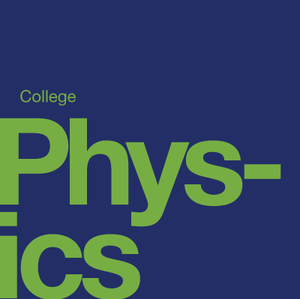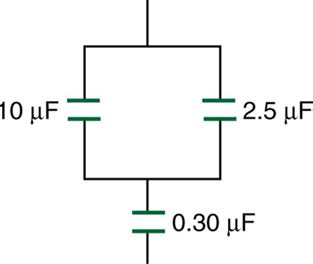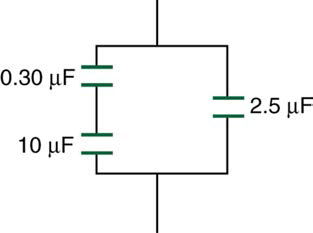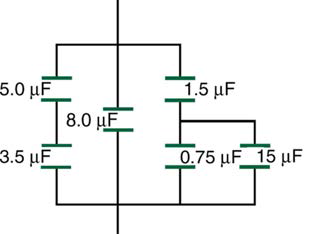# Electric Potential and Electric Field

Source: OpenStax

Student Price: FREE

This pack examines potential energy and potential difference, potential in a uniform field, point charges, equipotential lines, capacitors and more.

PE 19.1

Find the ratio of speeds of an electron and a negative hydrogen ion (one having an extra electron) accelerated through the same voltage, assuming non-relativistic final speeds. Take the mass of the hydrogen ion to be 1.67×10$^{-27}$ kg.

PE 19.2

An evacuated tube uses an accelerating voltage of 40 kV to accelerate electrons to hit a copper plate and produce x rays. Non-relativistically, what would be the maximum speed of these electrons? (answer in ×10$^{8}$ m/s)

PE 19.4

Integrated concepts Singly charged gas ions are accelerated from rest through a voltage of 13.0 V. At what temperature will the average kinetic energy of gas molecules be the same as that given these ions? (answer in ×10$^{5}$ K)

PE 19.5

Integrated concepts The temperature near the center of the Sun is thought to be 15 million degrees Celsius (1.5×10$^{7}$ ºC). Through what voltage must a singly charged ion be accelerated to have the same energy as the average kinetic energy of ions at this temperature? (answer in ×10$^{3}$ V)

PE 19.9

Integrated concepts A battery-operated car utilizes a 12.0 V system. Find the charge the batteries must be able to move in order to accelerate the 750 kg car from rest to 25.0 m/s, make it climb a 2.00×10$^{2}$ m high hill, and then cause it to travel at a constant 25.0 m/s by exerting a 5.00×10$^{2}$ N force for an hour. (answer in ×10$^{6}$ C)

PE 19.14

What is the strength of the electric field between two parallel conducting plates separated by 1.00 cm and having a potential difference (voltage) between them of 1.50×10$^{4}$ V ? (answer in ×10$^{6}$ V/m)

PE 19.16

How far apart are two conducting plates that have an electric field strength of 4.50×10$^{3}$ V/m between them, if their potential difference is 15.0 kV? (answer in m)

PE 19.18

The voltage across a membrane forming a cell wall is 80.0 mV and the membrane is 9.00 nm thick. What is the electric field strength? (The value is surprisingly large, but correct. Membranes are discussed in Capacitors and Dielectrics and Nerve Conduction-Electrocardiograms.) You may assume a uniform electric field. (answer in ×10$^{6}$ V/m)

PE 19.19

Membrane walls of living cells have surprisingly large electric fields across them due to separation of ions. (Membranes are discussed in some detail in Nerve Conduction-Electrocardiograms.) What is the voltage across an 8.00 nm-thick membrane if the electric field strength across it is 5.50 MV/m? You may assume a uniform electric field. (answer in mV)

PE 19.21

Find the maximum potential difference between two parallel conducting plates separated by 0.500 cm of air, given the maximum sustainable electric field strength in air to be 3.0 ×10$^{6}$ V/m . (answer in kV)

PE 19.22

A doubly charged ion is accelerated to an energy of 32.0 keV by the electric field between two parallel conducting plates separated by 2.00 cm. What is the electric field strength between the plates? (answer in ×10$^{5}$ V/m

PE 19.24

A 0.500 cm diameter plastic sphere, used in a static electricity demonstration, has a uniformly distributed 40.0 pC charge on its surface. What is the potential near its surface? (answer in V)

PE 19.25

What is the potential 0.530×10$^{-10}$ m from a proton (the average distance between the proton and electron in a hydrogen atom)? (answer in V)

PE 19.28

What are the sign and magnitude of a point charge that produces a potential of -2.00 V at a distance of 1.00 mm? (answer in ×10$^{-13}$ C)

PE 19.46

What charge is stored in a 180 µF capacitor when 120 V is applied to it? (answer in mC)

PE 19.47

Find the charge stored when 5.50 V is applied to an 8.00 pF capacitor. (answer in pC)

PE 19.48

What charge is stored in the capacitor in Example 19.8? (answer in mC)

PE 19.49

Calculate the voltage applied to a 2.00 µF capacitor when it holds 3.10 µC of charge. (answer in V)

PE 19.50

What voltage must be applied to an 8.00 nF capacitor to store 0.160 mC of charge? (answer in kV)

PE 19.51

What capacitance is needed to store 3.00 µC of charge at a voltage of 120 V? (answer in nF)

PE 19.52

What is the capacitance of a large Van de Graaff generator's terminal, given that it stores 8.00 mC of charge at a voltage of 12.0 MV? (answer in pF)

PE 19.53

Find the capacitance of a parallel plate capacitor having plates of area 5.00 m$^2$ that are separated by 0.100 mm of Teflon. (answer in μF)

PE 19.57Find the total capacitance of the combination of capacitors in Figure. (answer in μF)

Figure: A combination of series and parallel connections of capacitors.

PE 19.58

Suppose you want a capacitor bank with a total capacitance of 0.750 F and you possess numerous 1.50 mF capacitors. What is the smallest number you could hook together to achieve your goal, and how would you connect them? (answer in capacitors)

PE 19.60Find the total capacitance of the combination of capacitors shown in Figure. (answer in μF) Figure: A combination of series and parallel connections of capacitors.

PE 19.61Find the total capacitance of the combination of capacitors shown in Figure. (answer in μF) Figure: A combination of series and parallel connections of capacitors.

PE 19.65

A 165 µF capacitor is used in conjunction with a motor. How much energy is stored in it when 119 V is applied? (answer in J)

CC BY - OpenStax